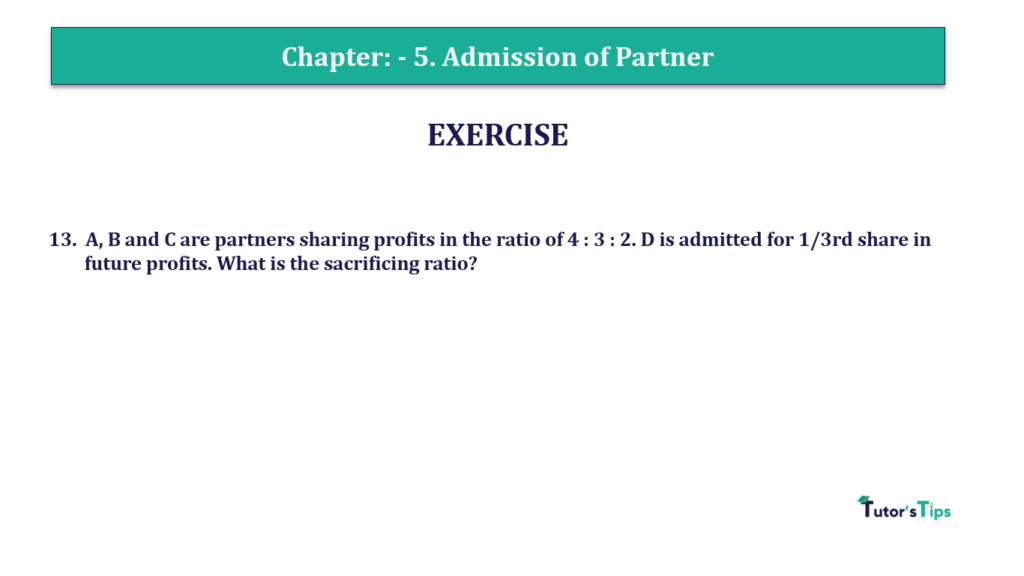# Question 13 Chapter 5 of +2-A – T.S. Grewal 12 Class Part – A Vol. 1Question 13 Chapter 5 of +2-A

13. A, B and C are partners sharing profits in the ratio of 4 : 3 : 2. D is admitted for 1/3rd share in future profits. What is the sacrificing ratio?

The solution of Question 13 Chapter 6 of +2-A

 Old Ratio of A, B and C = 4 : 3 : 2 A is admitted for 1/3rd share of profit

Let the total share of the business = 1
Remaining share of A, B and C  = Total Share – D’s Share

 Remaining share = 1 – 1 3
 = 3 – 1 3
 = 2 3

To Calculate to New Ratio distribute the remaining share in the old ratio of old partners’

New Ratio = Combined share of A, B and C X Old Ratio

 A’s New Ratio = 2 X 4 3 9
 = 8 27

 B’s New Ratio = 2 X 3 3 9
 = 6 27

 C’s New Ratio = 2 X 2 3 9
 = 4 27

 D’s New Ratio = 1 X 9 3 9
 = 9 27

 New Profit sharing Ratio between A, B, C, and D = 8 : 6 : 4 : 9

Calculation of Sacrificing Ratio

 Old Ratio of A, B and C = 4 : 3 : 2 New Ratio of A, B, and C = 8 : 6 : 4 : 9

Sacrificing Share = Old Ratio – New Ratio

 A’s Sacrificing Share = 4 – 8 9 27
 = 12 – 8 27
 = 4 27

 B’s Sacrificing Share = 3 – 6 9 27
 = 9 – 6 27
 = 3 27

 A’s Sacrificing Share = 2 – 4 9 27
 = 6 – 4 27
 = 2 27
 Sacrificing Share = 4 : 3 : 2

### T.S. Grewal’s Double Entry Book Keeping +2 (Vol. I: Accounting for Not-for-Profit Organizations and Partnership Firms)

• Chapter No. 1 – Financial Statement of Not-For-Profit Organisations
• Chapter No. 2 – Accounting for Partnership Firms – Fundamentals
• Chapter No. 3 – Goodwill: Nature and Valuation
• Chapter No. 4 – Change in Profit-Sharing Ratio Among the Existing Partners
• Chapter No. 5 – Admission of a Partner
• Chapter No. 6 – Retirement/Death of a Partner
• Chapter No. 7 – Dissolution of a Partnership Firm

### T.S. Grewal’s Double Entry Book Keeping (Vol. II: Accounting for Companies)

• Chapter No. 1 – Financial Statements of a Company
• Chapter No. 2 – Financial Statement Analysis
• Chapter No. 3 – Tools of Financial Statement Analysis – Comparative Statements and Common- Size Statements
• Chapter No. 4 – Accounting Ratios
• Chapter No. 5 – Cash Flow Statement